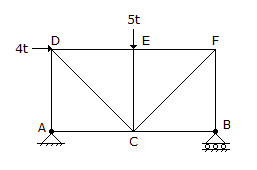# Civil Engineering - Theory of Structures - Discussion

Discussion Forum : Theory of Structures - Section 1 (Q.No. 38)
38.
The force in AD of the truss shown in given figure, is4.0t compression
3.0t compression
0.5t compression
0.5t tension
zero.
Explanation:
No answer description is available. Let's discuss.
Discussion:
9 comments Page 1 of 1.

Pawan said:   4 years ago
Thanks @TAPAS.

Very good, thanks @Dhendu.

Tapas said:   5 years ago
@Manoj Paridwal.

When axial force moves towards the joint it is compression. As the reaction on the left side +ve so it is an upward reaction, so to balance it an equal downward force will be induced in the member AD. Hence both as the forces are moving towards the joint A hence the member AD is under compression.

Manoj paridwal said:   6 years ago
How it is compression. Show it will be .5 tension?

Michelle said:   7 years ago
Hi @Ddendu.

How is EF=BC= X? Are all the members equal in a truss all the time?

Pradyumna Kumar Nayak said:   7 years ago
Considering the joint A.
As Va = 0.5 T upward.

fac = 0 & fad = 0.5T compression.

Pradyumna Kumar Nayak said:   8 years ago
Hello, Ishan.

It is a perpendicular distance of line of force and point B. So only x is multiplied.

Ishan said:   8 years ago
Hello Ddendu can you tell me, why the x is multiplied with 4t, as it is 2x distance away from B.

Reactions are Va + Vb = 5t.

Let length of each member be x.

Moment atB = 4t * x - 5t * x + Va * 2x = 0.

Therefore 1t = 2Va.

Hence Va = 0.5t.
(1)